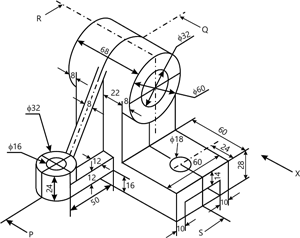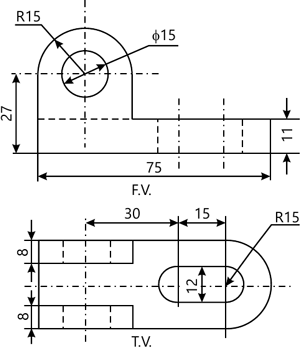MORE IN Engineering Graphics
GTU First Year Engineering (Semester 2)
Engineering Graphics
June 2014
Total marks: --
Total time: --
INSTRUCTIONS
(1) Assume appropriate data and state your reasons
(2) Marks are given to the right of every question
(3) Draw neat diagrams wherever necessary

Choose the correct altenative:
1 (a) (i) When the plane cuts the cone parallel to the generator, the curve traced out is
(a)ellipse (b) parabola (c) hyperbola (d) triangle
1 M
1 (a) (ii) When a line is inclined to VP and parallel to HP, the front view will be _______ to xy.
(a) parallel (b) perpendicular (c) inclined at angle (d) none of these
1 M
1 (a) (iii) A square plate of negligible thickness is inclined to HP. The front view will appear as
(a) rhombus (b) square (c) line (d) rectangle
1 M
1 (a) (iv) Name the solid formed by four equilateral triangle
(a)Square pyramid (b) Triangular pyramid (c) Tetrahedron (d) Square prism
1 M
1 (a) (v) A cylinder standing on the HP is cut by a vertical plane parallel to the axis and away from it. The shape of the section will be
(a) Rectangle (b) Circle (c) Ellipse (d) Hyperbola
1 M
1 (a) (vi) Two lines inclined at 90?? in the orthographic view appear in isometric view to be inclined at?
(a) 60?? (b) 90?? (c) 120?? (d) 180??
1 M
1 (a) (vii) When the axis of the solid is parallel to both HP and VP the view which reveals the true shape of the base is
(a) Front view (b) Top view (c) Side view (d) None of these
1 M
1 (b) (i) Length of a line L in isometric drawing or view will be ?
(a)0.707 L (b) 0.815 L (c) 0.866 L (d) equal to length L
1 M
1 (b) (ii) If D is the diameter of sphere, its value in isometric projection will be equal to
[(a) sqrt{3/2}D \ (b) sqrt{2/3}D \ (c) D none of these ]
1 M
1 (b) (iii) For drawing of small instruments, watches etc. the scale used is
(a) Reduced scale (b) Full scale (c) Enlarged scale (d) none of these
1 M
1 (b) (iv) Second angle projection is not used because
(a) Plan is above xy
(b) both views overlap each other
(c) elevation is above xy
(d) views are small in size
1 M
1 (b) (v) If the object lies in third quadrant, its position with respect to reference planes will be
(a) In front of VP, above HP
(b) Behind VP, above HP
(c) Behind VP, below HP
(d) In front of VP, below HP
1 M
1 (b) (vi) In the game of cricket, a ball is thrown from the boundary and reaches the gloves of the wicket keeper, the curve traced out will be
(a) Hyperbola (b) Involute (c) Parabola (d) Cycloid
1 M
1 (b) (vii) In isometric projection/drawing the ellipse is normally drawn by which method
(a) Arc of circle method (b) Concentric circle method (c) Four centre method (d) Oblong method
1 M

2 (a) Draw an Archemedian spiral of 1.5 convolutions, the greatest and least radii being 125 mm and 35 mm respectively. Draw tangent and normal to the spiral at any point on the curve.
7 M
2 (b) Construct a plain scale with RF = 1/5 to show decimeters (dm) and centimeters. The scale should be long enough to measure 1 m. Show the length of 7.4 dm on it.
7 M

3 (a) A thin 30°-60° set square has its longest edgeis 80 mm in the VP and inclined at 45° to the HP. Its surface makes an angle of 60° with the VP. Draw its projections.
7 M
3 (b) A cube of 50 mm long edges has its vertical faces equally inclined to VP. It is cut by a section plane perpendicular to VP so that the true shape of the section is a regular hexagon. Determine the inclination of the cutting plane with the HP and draw the sectional top view and true shape of the section
7 M

4 (a) A line AB 90 mm long is inclined at 30° to the HP. Its end A is 12 mm above the HP and 20 mm in front of VP. Its front view measures 65 mm. Draw the top view of AB and state its length. Determine the inclination of top view and line AB withVP.
7 M
4 (b) A hexagonal pyramid, side of the base 25 mm long and height 70 mm resting on HP on its side, has one of its triangular faces perpendicular to the HP and inclined at 60°to VP. Draw its projections.
7 M

5 (a) A line AB measures 80 mm in top view and 70 mm in front view. The midpoint M of the line is 45 mm in front of VP and 35 mm above HP. The end A is 10 mm in front of VP. Draw the rojections of the line and find its true length and inclination with HP and VP
7 M
5 (b) A square lamina of side 35 mm is resting on HP on one of its sides such that the surface of the lamina is inclined at 40° to HP. Draw its top view and front view when the side on which it rests is parallel to VP.
7 M

6 Figure 1 shows an object. Draw sectional front view along section P-Q looking inthe direction of arrow X , top view and sectional left hand side view along section R-S using first angle projection method.14 M

7 Figure 2 shows the front view and top view of an object. Draw the isometric view.14 M

More question papers from Engineering Graphics